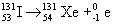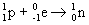# 21.S: Nuclear Chemistry (Summary)

$$\newcommand{\vecs}{\overset { \rightharpoonup} {\mathbf{#1}} }$$ $$\newcommand{\vecd}{\overset{-\!-\!\rightharpoonup}{\vphantom{a}\smash {#1}}}$$$$\newcommand{\id}{\mathrm{id}}$$ $$\newcommand{\Span}{\mathrm{span}}$$ $$\newcommand{\kernel}{\mathrm{null}\,}$$ $$\newcommand{\range}{\mathrm{range}\,}$$ $$\newcommand{\RealPart}{\mathrm{Re}}$$ $$\newcommand{\ImaginaryPart}{\mathrm{Im}}$$ $$\newcommand{\Argument}{\mathrm{Arg}}$$ $$\newcommand{\norm}{\| #1 \|}$$ $$\newcommand{\inner}{\langle #1, #2 \rangle}$$ $$\newcommand{\Span}{\mathrm{span}}$$ $$\newcommand{\id}{\mathrm{id}}$$ $$\newcommand{\Span}{\mathrm{span}}$$ $$\newcommand{\kernel}{\mathrm{null}\,}$$ $$\newcommand{\range}{\mathrm{range}\,}$$ $$\newcommand{\RealPart}{\mathrm{Re}}$$ $$\newcommand{\ImaginaryPart}{\mathrm{Im}}$$ $$\newcommand{\Argument}{\mathrm{Arg}}$$ $$\newcommand{\norm}{\| #1 \|}$$ $$\newcommand{\inner}{\langle #1, #2 \rangle}$$ $$\newcommand{\Span}{\mathrm{span}}$$$$\newcommand{\AA}{\unicode[.8,0]{x212B}}$$

• nucleons – neutron and proton
• all atoms of a given element have the same number of protons, atomic number
• isotopes – atoms with the same atomic number but different mass numbers
• three isotopes of uranium: uranium-233, uranium-235, uranium-238
•(superscript is mass number, subscript atomic number)

### 21.1.1 Nuclear Equations

• alpha particles – helium-4 particles
• alpha radiation – stream of alpha particles
• emission of radiation is one way that an unstable nucleus is transformed into a more stable one
•• superscript = mass number
• subscript = atomic number
• radioactive decay – when a nucleus spontaneously decomposes
• sum of the mass numbers is the same on both sides of the equation
• sum of the atomic numbers same on both sides of the equation
• radioactive properties of the nucleus are independent of the state of chemical combination of the atom
• chemical form does not matter when writing nuclear equations

### 21.1.2 Types of Radioactive Decay

• three most common type of radioactive decay: alpha(α), beta(β), and gamma(γ) radiation
Property α β γ
Charge 2+ 1- 0
Mass 6.64x10-24 g 9.11x10-28 g 0
Relative penetrating power 1 100 10,000
Nature of radiation $$\ce{^{2}_4 He} \, \text{nuclei}$$ electrons High-energy photons
• beta particles – high speed electrons emitted by an unstable nucleus
•• beta decay results in increasing the atomic number
•• gamma radiation – high-energy protons
• gamma radiation does not change atomic number or mass number or a nucleus
• almost always accompanies other radioactive emission
• represents the energy lost when the remaining nucleons reorganize into more stable arrangements
• positron – particle that has same mass as an electron but opposite charge
• represented by• emission of a positron has effect of converting a proton to a neutron decreasing atomic number of nucleus by 1
• electron capture – the capture by the nucleus of an inner-shell electron from the electron cloud surrounding the nucleus
• has effect of converting a proton to neutron
•Particle Symbol
Neutron $$\ce{^{1}_0n}$$
Proton $$\ce{^{1}_1H}$$ or $$\ce{^{1}_1p}$$
Electron $$\ce{^{0}_{-1}e}$$
Alpha Particle $$\ce{^{4}_2 He}$$ or $$\ce{^{4}_2 \alpha}$$
Beta Particle $$\ce{^{0}_{-1} e}$$ or $$\ce{^{0}_{-1} \beta}$$
Positron $$\ce{^{0}_1e}$$

## 21.2: Patterns of Nuclear Stability

### 21.2.1 Neutron-to-Proton Ratio

• strong nuclear force – a strong force of attraction between a large number of protons in the small volume of the nucleus
• stable nuclei with low atomic numbers up to 20 have nearly equal number of neutrons and protons
• for higher atomic numbers, the number of neutrons are greater than the number of protons
• the neutron-to-proton ratio of stable nuclei increase with increasing atomic number
• belt of stability – area where all stable nuclei are found
• ends at bismuth
• all nuclei with 84 or more protons are radioactive
• an even number of protons and neutrons is more stable than an odd number
• determining type of radioactive decay
• 1) nuclei above the belt of stability
• high neutron-to-proton ratios
• move toward belt of stability by emitting a beta particle
• decreases the number of neutrons and increases the number of protons in a nucleus
• 2) nuclei below the belt of stability
• low neutron-to-proton ratios
• move toward belt of stability by positron emission or electron capture
• increase number of neutrons and decrease the number of protons
• positron emission more common with lower nuclear charges
• electron capture becomes more common with increasing nuclear charge
• 3) nuclei with atomic numbers84
• alpha emission
• decreases both number of neutrons and protons by 2

• some nuclei cannot game stability by a single emission
• radioactive series or nuclear disintegration series – series of nuclear reactions that begin with an unstable nucleus to a stable one
• three types of radioactive series found in nature

### 21.2.3 Further Observations

• nuclei with 2, 8, 20, 28, 50, or 82 protons or 2, 8, 20, 28, 50, 82, or 126 neutrons are more stable than with nuclei without these numbers
• numbers called magic numbers
• nuclei with even number of protons and neutrons more stable than with odd number of protons and neutrons
• observations made in terms of the shell model of the nucleus
• nucleons reside in shells
• magic numbers represent closed shells in nuclei

## 21.3: Nuclear Transmutations

• nuclear transmutations – nuclear reactions caused by the collision of one nucleus with a neutron or by another nucleus
• first conversion of one nucleus into another performed by Ernest Rutherford in 1919
• converted nitrogen-14 to oxygen-17
••### 21.3.1 Using Charged Particles

• particle accelerators – used to accelerate particles at very high speeds
• cyclotron, and synchrotron

### 21.3.2 Using Neutrons

• neutrons do not need to be accelerated

### 21.3.4 Transuranium Elements

• transuranium elements – elements with atomic numbers above 92 that are produced by artificial transmutations

## 21.4: Rates of Radioactive Decay

• radioactive decay is a first-order process
• has characteristic of half life, which is the time required for half of any given quantity of a substance to react
• half-life unaffected by external conditions

### 21.4.1 Dating

• radiocarbon dating assumes that the ratio of carbon-14 to carbon-12 in the atmosphere has been constant for at least 50,000 years
• age of rocks can be determined by ratio of uranium-238 to lead-206

### 21.4.2 Calculations Based on Half-life

• rate = kN
• $$k$$ = decay constant, N = nuclei
• $\ln \dfrac{N_t}{N_o} = -k t \nonumber$
• t = time interval of decay, k = decay constant, N0 = initial number of nuclei at time zero, Nt = number remaining after time interval
• $k= \dfrac{0.693}{t_{1/2}} \nonumber$

• Geiger counter – device used to measure and detect radioactivity
• Based on ionization of matter caused by radiation
• Phosphors – substances that give off light when exposed to radiation
• Scintillation counter – used to detect and measure radiation based on tiny flashes of light produced when radiation strikes a suitable phosphor

• radioisotopes can be used to follow an element through its chemical reactions
• isotopes of same element have same properties

## 21.6: Energy Changes in Nuclear Reactions

$E=mc^2 \nonumber$

E = energy, m = mass, c = speed of light

If system loses mass, it loses energy (exothermic)

If system gains mass, it gains energy (endothermic)

### 21.6.1 Nuclear Binding Energies

• masses of nuclei always less than masses of individual nucleons
• mass defect – mass difference between a nucleus and its constituent nucleons
• energy is needed to break nucleus into separated protons and neutrons, addition of energy must also have an increase in mass
• nuclear binding energy – energy required to separate a nucleus into its individual nucleons
• the larger to nuclear binding energy the more stable the nucleus toward decomposition
• fission – energy produced when heavy nuclei split
• fusion – energy produced when light nuclei fuse

## 21.7: Nuclear Fission

• fission and fusion both exothermic
• chain reaction – reaction in which the neutrons produced in one fission cause further fission reactions
• in order for a fission chain reaction to occur, the sample of fissionable material must have a certain minimum mass
• critical mass – amount of fissionable material large enough to maintain the chain reaction with a constant rate of fission
• supercritical mass – mass in excess of a critical mass

### 21.7.1 Nuclear Reactors

• nuclear reactors the fission is controlled to generate a constant power
• reactor core consists of fissionable fuel, control rods, a moderator, and cooling fluid
• fission products are extremely radioactive and are thus hard to store
• about 20 half-lives needed for products to react acceptable levels for biological exposure

### 21.8: Nuclear Fusion

• fusion is appealing because of availability of light isotopes and fusion products are not radioactive
• high energies needed to overcome attraction of nuclei
• thermonuclear reactions – fusion reactions
• lowest temperature required is about 40,000,000 K

## 21.9: Biological Effects of Radiation

• when matter absorbs radiation, the energy of the radiation can cause either excitation or ionization
• most of energy of radiation absorbed by water molecules
• free radical – a substance with one ore more unpaired electrons
• can attack other biomolecules to produce more free radicals
• gamma rays most dangerous
• tissues that take most damage are the ones that reproduce at a rapid rate
• bone marrow, blood forming tissues, lymph nodes

• becquerel (Bq) – SI unit for activity of the radiation source; rate at which nuclear disintegrations are occurring
• 1 (Bq) = 1 nuclear disintegration/s
• curie (Ci) = 3.7x1010 disintegrations/s = rate of decay of 1g of radium
• two units used to measure amount of exposure to radiation: gray (Gy) and rad
• gray – SI unit of absorbed dose = absorption of 1 J of energy per kilogram of tissue
• rad (radiation absorbed dose) – absorption of 1x10-2 J of energy per kilogram of tissue
• 1 Gy = 100 rads
• relative biological effectiveness – RBE
• exact value varies with dose rate, total dose, and type of tissue affected
• rem (roentgen equivalent for man) – product of the radiation dose in rads and the RBE of the radiation gibes the effective dosage
• rem is unit of radiation damage that is usually used in medicine
• number of rems = (number of rads)(RBE)
• Sievert (Sv) – SI unit for dosage
• 1 Sv = 100 rem
• annual exposure = 360mrem